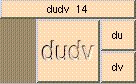DUDVTHE BOX'S MAIN HELP: This vector represents the rate of change in the surface with respect to u and v. A vector is returned only for free-form surface objects, for polygonal objects the vector is null. du (output): This vector represents the rate of change in the surface with respect to u. A vector is returned only for free-form surface objects, for polygonal objects the vector is null. dv (output): This vector represents the rate of change in the surface with respect to v. A vector is returned only for free-form surface objects, for polygonal objects the vector is null.

<-- BACK TO Math